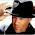## Monday, December 13, 2021

### Exersing Python 3.10 on Fedora 25 via sorting Pandas DataFrame

The following below is a not entirely trivial algorithm for students that creates a Python dictionary when scanning text according to the conditions of the problem, which is then processed as Pandas Dataframe to provide the output required

The original problem itself

The text file alpha-wide.txt contains only capital letters of the Latin alphabet (ABC… Z). Identify the character that most often occurs in the file immediately after the letter X. In the answer first write down this character, and then separate by blank how many times it occurred after letters X. If there are several such characters, you need to display the one that appears earlier in the alphabet.

The file alpha-wide.txt contains a pretty long string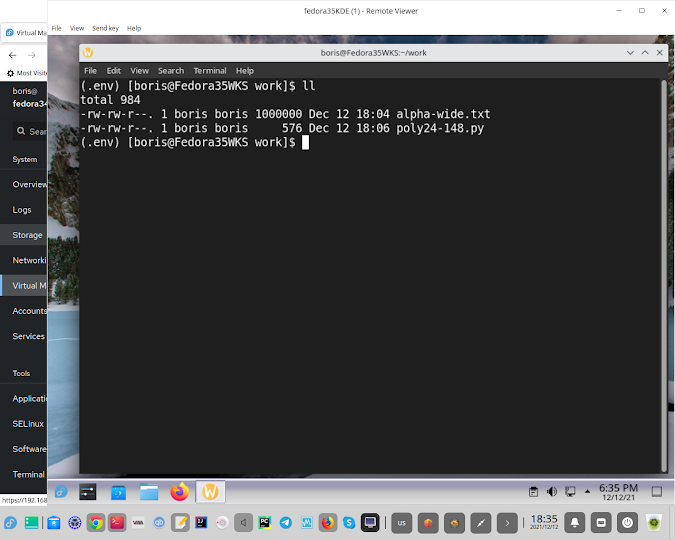Python code has been set up in a virtual environment with pandas installed via pip

import pandas as pd

with open("./alpha-wide.txt") as F:

string = F.readline() # getting next line

d={} # declare dictionary of letters

while "X" in string:

n = string.find("X") # index "X"

key = string[n+1]    # create key = Letter

d[key] = d.get(key,0)+1

string = string[n+1:] # slice, form a new line

# print(d)

data_items = d.items()

data_list = list(data_items)

df = pd.DataFrame(data_list,columns = ['X1','Y1'])

df.sort_values("Y1", axis = 0, ascending = True, inplace = True, na_position ='last')

print("DataFrame has been sorted by Y1")

print(df)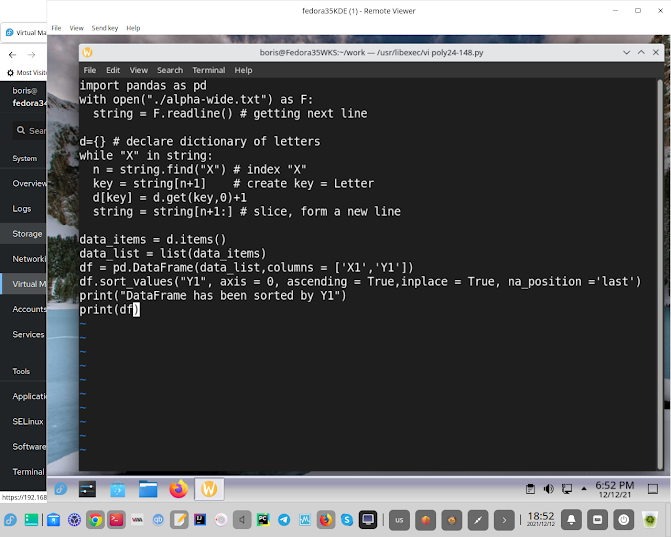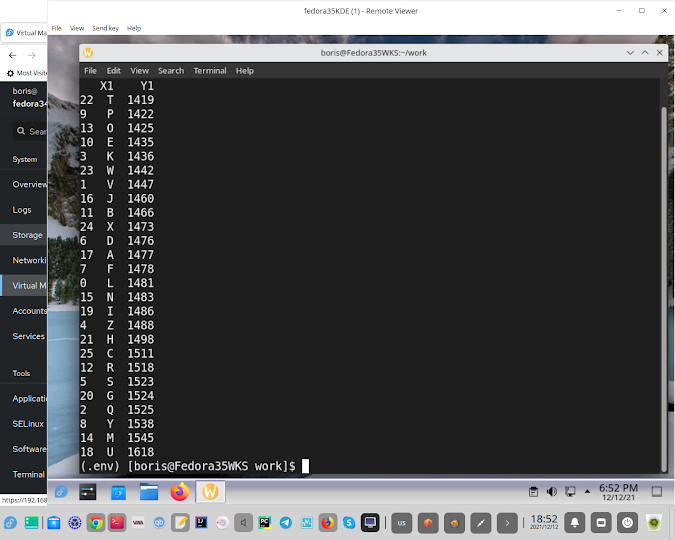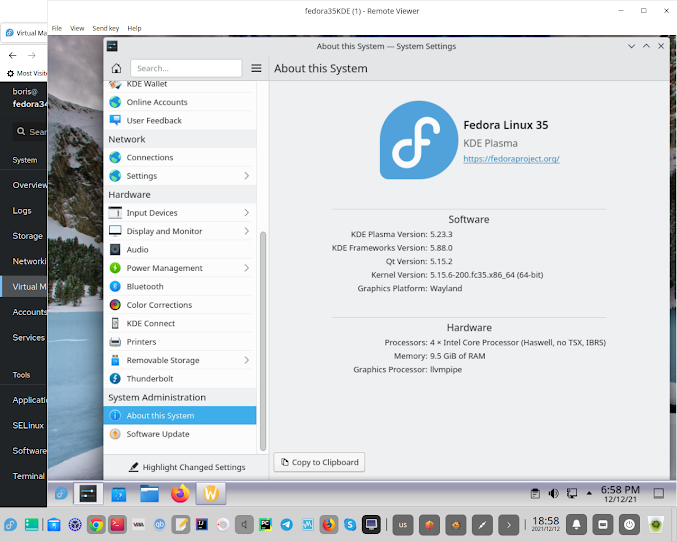1.2.